#Function Repository Resource:

# SecDegree

Computes the secant of an angle given in degrees

Contributed by: Paco Jain (Wolfram Research)
 ResourceFunction["SecDegree"][x] computes the secant of an angle x, measured in degrees

## Details and Options

Mathematical function, suitable for both symbolic and numerical manipulation.
For certain special arguments, SecDegree automatically evaluates to exact values.
SecDegree can be evaluated to arbitrary numerical precision.
SecDegree automatically threads over lists.

## Examples

### Basic Examples

Compute the secant of an angle:

 In:=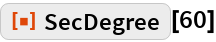Out=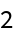Plot over a given range of angles:

 In:=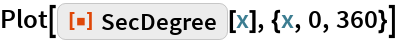Out=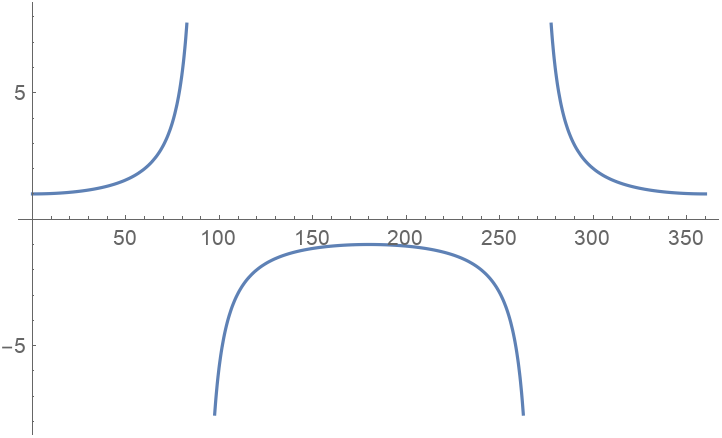### Scope

Evaluate numerically:

 In:=Out=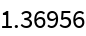Evaluate to high precision:

 In:=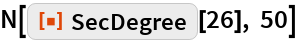Out=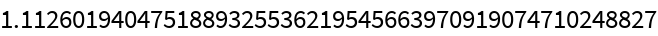## Requirements

Wolfram Language 11.3 (March 2018) or above